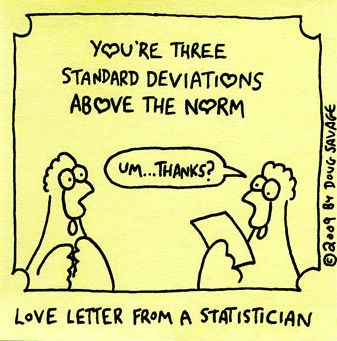Learn the 4 Things You Must Know Before Taking the GMAT!   Download it Now!

# How Variance is Tested on the GMATLet’s start with the good news: There’s not a whole lot of statistics tested on the GMAT.

Here’s the even better news: The statistics that is tested doesn’t require intricate knowledge of complex formulas; a broad understanding of the most important concepts is often enough to answer most questions.

One of those concepts is Variance.*

### Understanding Variance

It’s difficult to understand Variance without also understanding Standard Deviation. They’re closely related and both express how spread out a set of numbers is. I said you don’t need to know complex statistics formulas on the GMAT, so let me try to explain standard deviation and variance using plain English. In fact, let’s broadly define those two terms now:

Standard Deviation: The measure of the spread of a set of numbers around the mean (average). A high standard deviation indicates that the data points are spread out over a large range of values; a low standard deviation indicates that the data points tend to be close to the mean.

Variance: A measure of how far spread out a set of numbers is. Specifically, the variance of a set of numbers is the average of the square of the difference between each measurement and the mean.

Now, before you freak out and yell at me for effectively giving you a formula for variance secretly disguised as an English sentence, let me say two things. First, you’re exactly right. That’s what I did. Second, don’t worry about it. You won’t need to calculate variance on the GMAT. (If you’d like to see how to actually calculate variance for your own edification, check out the short video about variance at the end of this article).

Here’s what you do need to know — How standard deviation and variance are related. You may have noticed that my definitions for standard deviation and variance are essentially the same. Both are a measure of the spread of a set of numbers around the mean. So how are they different? Here is a formula that is worth you knowing for the GMAT that clearly and simply expresses the relationship between standard deviation and variance:

### Standard Deviation = √ (Variance)

It’s as simple as that. The standard deviation of a set of numbers is simply the square root of those numbers’ variance.

For example, if the variance of a set of numbers is 16, the standard deviation = √16 = 4. If the variance of a set of numbers is 9, then the standard deviation is 3. And so on. And of course you can go the other direction as well. If I tell you that the standard deviation of a set of numbers is 2, then you know that the variance of that same set of numbers = 2² = 4.

### Variance on the GMAT

So what does all this mean? What use does understanding variance have on the GMAT?

Apply what you’ve just learned and try your hand at this sample GMAT data sufficiency question from the GMAT Review Official Guide, 12th ed.:

Is the standard deviation of the set of measurements x₁, x₂, x₃, x₄, …, x₂₀  less than 3?

(1) The variance for the set of measurements is 4.
(2) For each measurement, the difference between the mean and that measurement is 2.

What may have formerly been a difficult question for you should now be as easy as pie given your newfound understanding of variance. Let’s evaluate each statement in turn:

Statement 1: If the variance is 4, then the standard deviation = √4 = 2, which is less than 3; SUFFICIENT.

Statement 2: For each measurement, the difference between the mean and that measurement is 2. Therefore, the square of each difference is 4, and the sum of all the squares is 4 * 20 = 80. The average of those differences is therefore 80 ÷ 20 = 4. Now you can calculate the standard deviation which is √4 = 2, which is less than 3; SUFFICIENT.